## Point-Point Distance--1-D

Given a unit Line Segment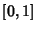, pick two points at random on it. Call the first pointand the second point. Find the distribution of distancesbetween points. The probability of the points being a (Positive) distanceapart (i.e., without regard to ordering) is given by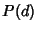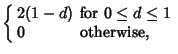(1)

whereis the Dirac Delta Function andis the Heaviside Step Function. The Moments are then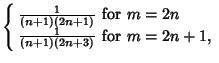(2)(3)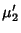(4)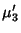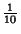(5)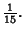(6)

The Moments can also be computed directly without explicit knowledge of the distribution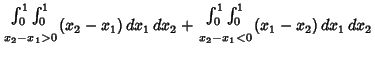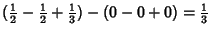(7)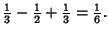(8)

The Moments about the Mean are therefore(9)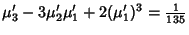(10)(11)

so the Mean, Variance, Skewness, and Kurtosis are(12)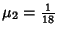(13)(14)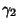(15)

The probability distribution of the distance between two points randomly picked on a Line Segment is germane to the problem of determining the access time of computer hard drives. In fact, the average access time for a hard drive is precisely the time required to seek across 1/3 of the tracks (Benedict 1995).